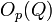# Local subgroup of finite group is contained in p-local subgroup for some prime p

Suppose$G$ is a finite group and$H$ is a local subgroup of$G$, i.e., it is the normalizer of some nontrivial solvable subgroup of$G$. Then, there exists a prime number$p$ and a p-local subgroup$K$ of$G$ such that$H \le K$.
Suppose$H = N_G(Q)$ for a solvable subgroup$Q$ of$G$. The idea is to find a prime$p$ such that$O_p(Q)$ (the p-core of$Q$) is nontrivial. Let$K = N_G(O_p(Q))$. We show that$H \le K$, completing the proof.